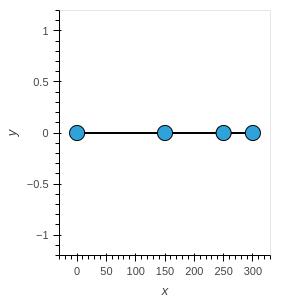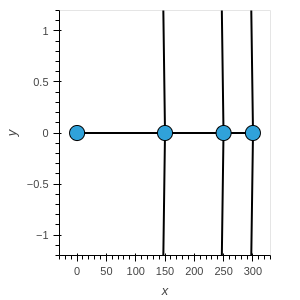# How to make a graph directed

Hello everybody,

I have some trouble making a network graph directed. This should be possible through the parameters ‘directed=True’. However, in my example below, adding ‘directed=True’ creates double the amount of arrows, which all lead nowhere, whereas without ‘directed=True’ the undirected graph is correctly displayed.
This is a saved png of undirected version (“Undirected.html” in the code below)and this the directed version (“Directed.html” in the code below) .If you have any ideas why this is or know where I went wrong, please let me know. Any help would be appreciated.

``````import numpy as np
import pandas as pd
import holoviews as hv
import networkx as nx
from holoviews import opts
from bokeh.models import HoverTool
import os
hv.extension('bokeh')

def nx_graph():
# create an example networkx graph with 4 nodes and edges connecting consecutive nodes
G = nx.Graph()
edge_list = [(1,2), (2,3), (3,4)]
for u,v in edge_list:
return G

def nx_to_hv(nx_ptn_graph):
# positions for the nodes
pos = {1:[0,0], 2:[150, 0], 3:[250, 0], 4:[300, 0]}
# convert to holoviews graph

def main():
# create a network x graph
G = nx_graph()
# convert it to a holoviews graph
undirected = nx_to_hv(nx_ptn_graph=G)
# save the undirected graph
hv.save(obj=undirected, filename="Undirected.html")
# make the hv graph directed
# save the result
hv.save(obj=directed, filename="Directed.html")
return

if __name__ == '__main__':
main()

``````

You are actually seeing the arrows: the underlying code appears to assume the positions of the vertices to be scaled. Try
` pos = {1:[0,0], 2:[1.50, 0], 3:[2.50, 0], 4:[3.00, 0]}`

1 Like

That works, thank you very muchA quick followup question: How would I fix that in general if I don’t know the coordinates ahead of time? What should I scale them with?

you would have to do what I did:
experiment, or try to find the code…

The provided layout codes all seem to work…

Alright, I’ll do that, thanks!

Any idea where I could find the scaling code? So far I had no luck in finding it, but I would like to build directed graphs that works with given node coordinates.